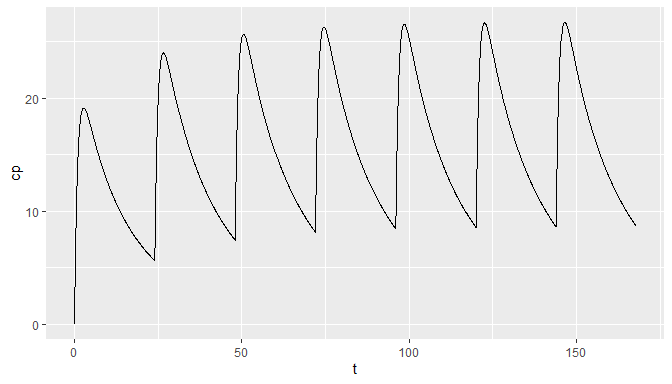# Drawing PK curves with pmxTools

library(pmxTools)
library(patchwork)

pmxTools includes functions for constructing pharmacokinetic (PK) concentration-time curves for a range of common compartmental systems using PK parameters and microconstants. The underlying solutions are based on Julie Bertrand and France Mentré’s Mathematical Expressions of the Pharmacokinetic and Pharmacodynamic Models implemented in the Monolix software, published in 2008.

The options available are by necessity confined to one-, two- and three-compartmental linear models with intravenous bolus, infusion or oral dosing (the latter with zero- or first-order absorption). If more complex systems are needed, you are probably better off using an ODE-based simulation package such as RxODE or mrgSolve.

## Specifying model parameters

Models take dose, time and PK parameters as inputs. Names for some PK parameters, especially volumes and intercompartmental clearances, vary by model type.

Parameter Description Used for
t Time Single-dose
tad Time after last dose Steady state
dose Dose All
CL Clearance All
V, V1 Central volume of distribution All
V2 First peripheral volume of distribution Two- and three-compartment models
V3 Second peripheral volume of distribution Three-compartment models
Q, Q1, Q2 Intercompartmental clearance (between V1 and V2) Two- and three-compartment models
Q3 Intercompartmental clearance (between V1 and V3) Three-compartment models
tlag Lag time Models with lag time
tinf Duration of infusion Models with infusion
dur Duration of zero-order absorption Models with zero-order absorption
ka First-order absorption rate Models with first-order absorption

## One-compartment, single dose

Available functions include:

Model Variant Call
1-compartment Single-dose, bolus calc_sd_1cmt_linear_bolus(t, dose, CL, V)
Single-dose, infusion calc_sd_1cmt_linear_infusion(t, dose, CL, V, tinf)
Single-dose, zero-order oral absorption calc_sd_1cmt_linear_oral_0(t, dose, CL, V, dur)
Single-dose, zero-order oral absorption with lag time calc_sd_1cmt_linear_oral_0_lag(t, dose, CL, V, dur, tlag)
Single-dose, first-order oral absorption calc_sd_1cmt_linear_oral_1(t, dose, CL, V, ka)
Single-dose, first-order oral absorption with lag time calc_sd_1cmt_linear_oral_1_lag(t, dose, CL, V, ka, tlag)

For example -


library(ggplot2)

t <- seq(0, 24, by=0.1)

df1 <- data.frame(t = t,
Cp1 = calc_sd_1cmt_linear_bolus(t = t, dose = 600, CL = 5, V = 20),
Cp2 = calc_sd_1cmt_linear_infusion(t = t, dose = 600, CL = 5, V = 20, tinf=2),
Cp3 = calc_sd_1cmt_linear_oral_0(t = t, dose = 600, CL = 5, V = 20, dur=2),
Cp4 = calc_sd_1cmt_linear_oral_0_lag(t = t, dose = 600, CL = 5, V = 20, dur=2, tlag=1),
Cp5 = calc_sd_1cmt_linear_oral_1(t = t, dose = 600, CL = 5, V = 20, ka=1.5),
Cp6 = calc_sd_1cmt_linear_oral_1_lag(t = t, dose = 600, CL = 5, V = 20, ka=1.5, tlag=1))

p1.1 <- ggplot(df1, aes(t, Cp1)) +
geom_line() +
labs(title="Bolus dose")

p1.2 <- ggplot(df1, aes(t, Cp2)) +
geom_line() +
labs(title="Infusion")

p1.3 <- ggplot(df1, aes(t, Cp3)) +
geom_line() +
labs(title="Zero-order oral")

p1.4 <- ggplot(df1, aes(t, Cp4)) +
geom_line() +
labs(title="Zero-order oral with lag time")

p1.5 <- ggplot(df1, aes(t, Cp5)) +
geom_line() +
labs(title="First-order oral")

p1.6 <- ggplot(df1, aes(t, Cp6)) +
geom_line() +
labs(title="First-order oral with lag time")

p1.1 + p1.2 + p1.3 + p1.4 + p1.5 + p1.6 + plot_layout(nrow=3)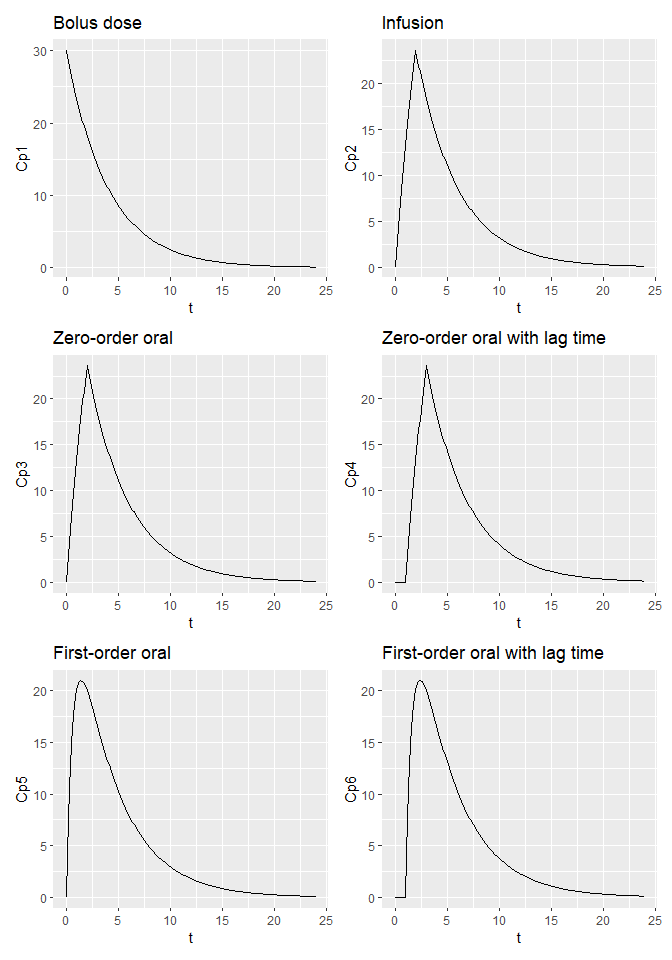Available functions include:

Model Variant Call
1-compartment Steady state, bolus calc_ss_1cmt_linear_bolus(tad, tau, dose, CL, V)
Steady state, infusion calc_ss_1cmt_linear_infusion(tad, tau, dose, CL, V, tinf)
Steady state, zero-order oral absorption calc_ss_1cmt_linear_oral_0(tad, tau, dose, CL, V, dur)
Steady state, zero-order oral absorption with lag time calc_ss_1cmt_linear_oral_0_lag(tad, tau, dose, CL, V, dur, tlag)
Steady state, first-order oral absorption calc_ss_1cmt_linear_oral_1(tad, tau, dose, CL, V, ka)
Steady state, first-order oral absorption with lag time calc_ss_1cmt_linear_oral_1_lag(tad, tau, dose, CL, V, ka, tlag)

For example -


t <- seq(0, 24, by=0.1)

df1ss <- data.frame(t = t,
Cp1 = calc_ss_1cmt_linear_bolus(t = t, tau = 24, dose = 600, CL = 1, V = 20),
Cp2 = calc_ss_1cmt_linear_infusion(t = t, tau = 24, dose = 600, CL = 1, V = 20, tinf=2),
Cp3 = calc_ss_1cmt_linear_oral_0(t = t, tau = 24, dose = 600, CL = 1, V = 20, dur=2),
Cp4 = calc_ss_1cmt_linear_oral_0_lag(t = t, tau = 24, dose = 600, CL = 1, V = 20, dur=2, tlag=1.5),
Cp5 = calc_ss_1cmt_linear_oral_1(t = t, tau = 24, dose = 600, CL = 1, V = 20, ka=1.5),
Cp6 = calc_ss_1cmt_linear_oral_1_lag(t = t, tau = 24, dose = 600, CL = 1, V = 20, ka=1.5, tlag=1.5))

p1.1ss <- ggplot(df1ss, aes(t, Cp1)) +
geom_line() +
labs(title="Bolus dose")

p1.2ss <- ggplot(df1ss, aes(t, Cp2)) +
geom_line() +
labs(title="Infusion")

p1.3ss <- ggplot(df1ss, aes(t, Cp3)) +
geom_line() +
labs(title="Zero-order oral")

p1.4ss <- ggplot(df1ss, aes(t, Cp4)) +
geom_line() +
labs(title="Zero-order oral with lag time")

p1.5ss <- ggplot(df1ss, aes(t, Cp5)) +
geom_line() +
labs(title="First-order oral")

p1.6ss <- ggplot(df1ss, aes(t, Cp6)) +
geom_line() +
labs(title="First-order oral with lag time")

p1.1ss + p1.2ss + p1.3ss + p1.4ss + p1.5ss + p1.6ss + plot_layout(nrow=3)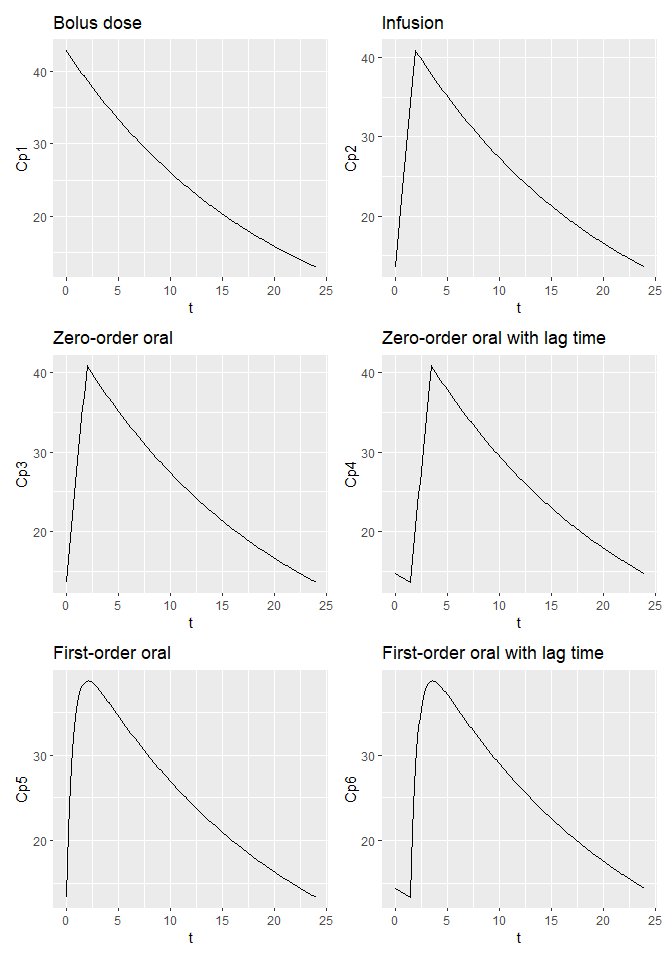## Two-compartment, single dose

Available functions include:

Model Variant Call
2-compartment Single-dose, bolus calc_sd_2cmt_linear_bolus(t, dose, CL, V1, V2, Q)
Single-dose, infusion calc_sd_2cmt_linear_infusion(t, dose, CL, V1, V2, Q, tinf)
Single-dose, zero-order oral absorption calc_sd_2cmt_linear_oral_0(t, dose, CL, V1, V2, Q, dur)
Single-dose, zero-order oral absorption with lag time calc_sd_2cmt_linear_oral_0_lag(t, dose, CL, V1, V2, Q, dur, tlag)
Single-dose, first-order oral absorption calc_sd_2cmt_linear_oral_1(t, dose, CL, V1, V2, Q, ka)
Single-dose, first-order oral absorption with lag time calc_sd_2cmt_linear_oral_1_lag(t, dose, CL, V1, V2, Q, ka, tlag)

For example -


t <- seq(0, 24, by=0.1)

df2 <- data.frame(t = t,
Cp1 = calc_sd_2cmt_linear_bolus(t = t, dose = 600, CL = 5, V1 = 20, V2 = 80, Q = 1),
Cp2 = calc_sd_2cmt_linear_infusion(t = t, dose = 600, CL = 5, V1 = 20, V2 = 80, Q = 1, tinf=2),
Cp3 = calc_sd_2cmt_linear_oral_0(t = t, dose = 600, CL = 5, V1 = 20, V2 = 80, Q = 1, dur=2),
Cp4 = calc_sd_2cmt_linear_oral_0_lag(t = t, dose = 600, CL = 5, V1 = 20, V2 = 80, Q = 1, dur=2, tlag=1),
Cp5 = calc_sd_2cmt_linear_oral_1(t = t, dose = 600, CL = 5, V1 = 20, V2 = 80, Q = 1, ka=1.5),
Cp6 = calc_sd_2cmt_linear_oral_1_lag(t = t, dose = 600, CL = 5, V1 = 20, V2 = 80, Q = 1, ka=1.5, tlag=1))

p2.1 <- ggplot(df2, aes(t, Cp1)) +
geom_line() +
labs(title="Bolus dose")

p2.2 <- ggplot(df2, aes(t, Cp2)) +
geom_line() +
labs(title="Infusion")

p2.3 <- ggplot(df2, aes(t, Cp3)) +
geom_line() +
labs(title="Zero-order oral")

p2.4 <- ggplot(df2, aes(t, Cp4)) +
geom_line() +
labs(title="Zero-order oral with lag time")

p2.5 <- ggplot(df2, aes(t, Cp5)) +
geom_line() +
labs(title="First-order oral")

p2.6 <- ggplot(df2, aes(t, Cp6)) +
geom_line() +
labs(title="First-order oral with lag time")

p2.1 + p2.2 + p2.3 + p2.4 + p2.5 + p2.6 + plot_layout(nrow=3)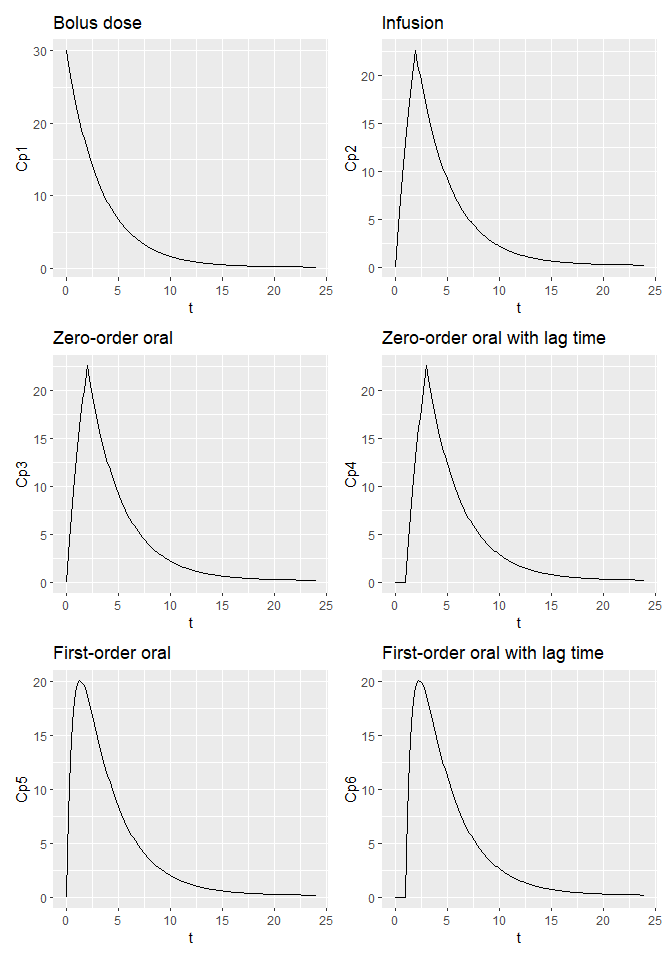Available functions include:

Model Variant Call
2-compartment Steady state, bolus calc_ss_2cmt_linear_bolus(tad, tau, dose, CL, V1, V2, Q)
Steady state, infusion calc_ss_2cmt_linear_infusion(tad, tau, dose, CL, V1, V2, Q, tinf)
Steady state, zero-order oral absorption calc_ss_2cmt_linear_oral_0(tad, tau, dose, CL, V1, V2, Q, dur)
Steady state, zero-order oral absorption with lag time calc_ss_2cmt_linear_oral_0_lag(tad, tau, dose, CL, V1, V2, Q, dur, tlag)
Steady state, first-order oral absorption calc_ss_2cmt_linear_oral_1(tad, tau, dose, CL, V1, V2, Q, ka)
Steady state, first-order oral absorption with lag time calc_ss_2cmt_linear_oral_1_lag(tad, tau, dose, CL, V1, V2, Q, ka, tlag)

For example -


t <- seq(0, 24, by=0.1)

df2ss <- data.frame(t = t,
Cp1 = calc_ss_2cmt_linear_bolus(tad = t, tau = 24, dose = 600, CL = 1, V1 = 20, V2 = 80, Q = 0.25),
Cp2 = calc_ss_2cmt_linear_infusion(tad = t, tau = 24, dose = 600, CL = 1, V1 = 20, V2 = 80, Q = 0.25, tinf=2),
Cp3 = calc_ss_2cmt_linear_oral_0(tad = t, tau = 24, dose = 600, CL = 1, V1 = 20, V2 = 80, Q = 0.25, dur=2),
Cp4 = calc_ss_2cmt_linear_oral_0_lag(tad = t, tau = 24, dose = 600, CL = 1, V1 = 20, V2 = 80, Q = 0.25, dur=2, tlag=1.5),
Cp5 = calc_ss_2cmt_linear_oral_1(tad = t, tau = 24, dose = 600, CL = 1, V1 = 20, V2 = 80, Q = 0.25, ka=1.5),
Cp6 = calc_ss_2cmt_linear_oral_1_lag(tad = t, tau = 24, dose = 600, CL = 1, V1 = 20, V2 = 80, Q = 0.25, ka=1.5, tlag=1.5))

p2.1ss <- ggplot(df2ss, aes(t, Cp1)) +
geom_line() +
labs(title="Bolus dose")

p2.2ss <- ggplot(df2ss, aes(t, Cp2)) +
geom_line() +
labs(title="Infusion")

p2.3ss <- ggplot(df2ss, aes(t, Cp3)) +
geom_line() +
labs(title="Zero-order oral")

p2.4ss <- ggplot(df2ss, aes(t, Cp4)) +
geom_line() +
labs(title="Zero-order oral with lag time")

p2.5ss <- ggplot(df2ss, aes(t, Cp5)) +
geom_line() +
labs(title="First-order oral")

p2.6ss <- ggplot(df2ss, aes(t, Cp6)) +
geom_line() +
labs(title="First-order oral with lag time")

p2.1ss + p2.2ss + p2.3ss + p2.4ss + p2.5ss + p2.6ss + plot_layout(nrow=3)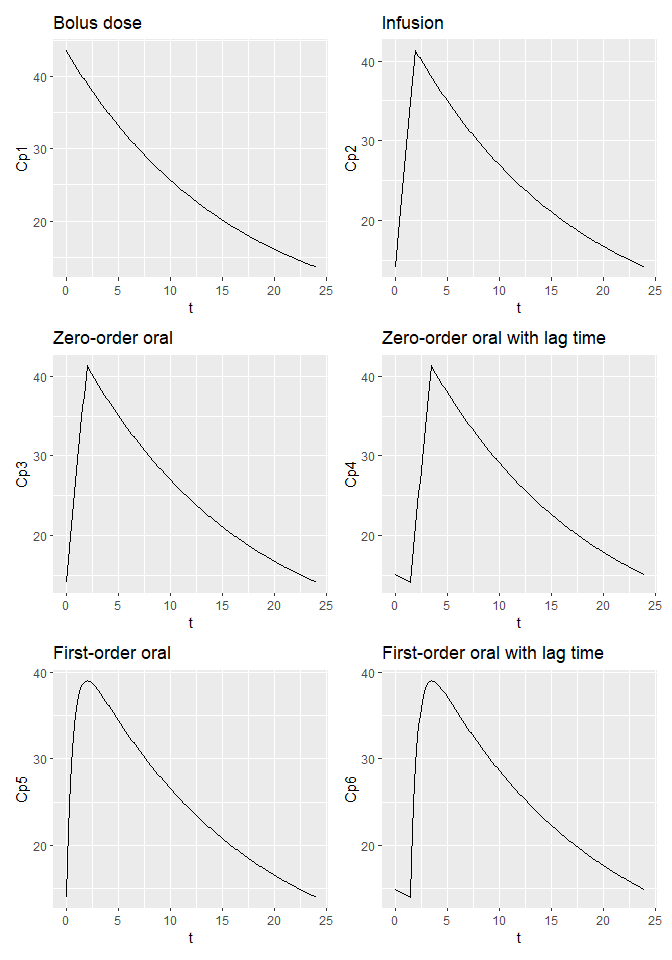## Three-compartment, single dose

Available functions include:

Model Variant Call
3-compartment Single-dose, bolus calc_sd_3cmt_linear_bolus(t, dose, CL, V1, V2, V3, Q2, Q3)
Single-dose, infusion calc_sd_3cmt_linear_infusion(t, dose, CL, V1, V2, V3, Q2, Q3, tinf)
Single-dose, zero-order oral absorption calc_sd_3cmt_linear_oral_0(t, dose, CL, V1, V2, V3, Q2, Q3, dur)
Single-dose, zero-order oral absorption with lag time calc_sd_3cmt_linear_oral_0_lag(t, dose, CL, V1, V2, V3, Q2, Q3, dur, tlag)
Single-dose, first-order oral absorption calc_sd_3cmt_linear_oral_1(t, dose, CL, V1, V2, V3, Q2, Q3, ka)
Single-dose, first-order oral absorption with lag time calc_sd_3cmt_linear_oral_1_lag(t, dose, CL, V1, V2, V3, Q2, Q3, ka, tlag)

For example -


t <- seq(0, 24, by=0.1)

df3 <- data.frame(t = t,
Cp1 = calc_sd_3cmt_linear_bolus(t = t, CL = 3.5, V1 = 20, V2 = 500, V3 = 200, Q2 = 0.5, Q3 = 0.05, dose = 600),
Cp2 = calc_sd_3cmt_linear_infusion(t = t, CL = 3.5, V1 = 20, V2 = 500, V3 = 200, Q2 = 0.5, Q3 = 0.05, dose = 600, tinf=2),
Cp3 = calc_sd_3cmt_linear_oral_0(t = t, CL = 3.5, V1 = 20, V2 = 500, V3 = 200, Q2 = 0.5, Q3 = 0.05, dose = 600, dur=2),
Cp4 = calc_sd_3cmt_linear_oral_0_lag(t = t, CL = 3.5, V1 = 20, V2 = 500, V3 = 200, Q2 = 0.5, Q3 = 0.05, dose = 600, dur=2, tlag=1),
Cp5 = calc_sd_3cmt_linear_oral_1(t = t, CL = 3.5, V1 = 20, V2 = 500, V3 = 200, Q2 = 0.5, Q3 = 0.05, dose = 600, ka=1.5),
Cp6 = calc_sd_3cmt_linear_oral_1_lag(t = t, CL = 3.5, V1 = 20, V2 = 500, V3 = 200, Q2 = 0.5, Q3 = 0.05, dose = 600, ka=1.5, tlag=1))

p3.1 <- ggplot(df3, aes(t, Cp1)) +
geom_line() +
labs(title="Bolus dose")

p3.2 <- ggplot(df3, aes(t, Cp2)) +
geom_line() +
labs(title="Infusion")

p3.3 <- ggplot(df3, aes(t, Cp3)) +
geom_line() +
labs(title="Zero-order oral")

p3.4 <- ggplot(df3, aes(t, Cp4)) +
geom_line() +
labs(title="Zero-order oral with lag time")

p3.5 <- ggplot(df3, aes(t, Cp5)) +
geom_line() +
labs(title="First-order oral")

p3.6 <- ggplot(df3, aes(t, Cp6)) +
geom_line() +
labs(title="First-order oral with lag time")

p3.1 + p3.2 + p3.3 + p3.4 + p3.5 + p3.6  + plot_layout(nrow=3)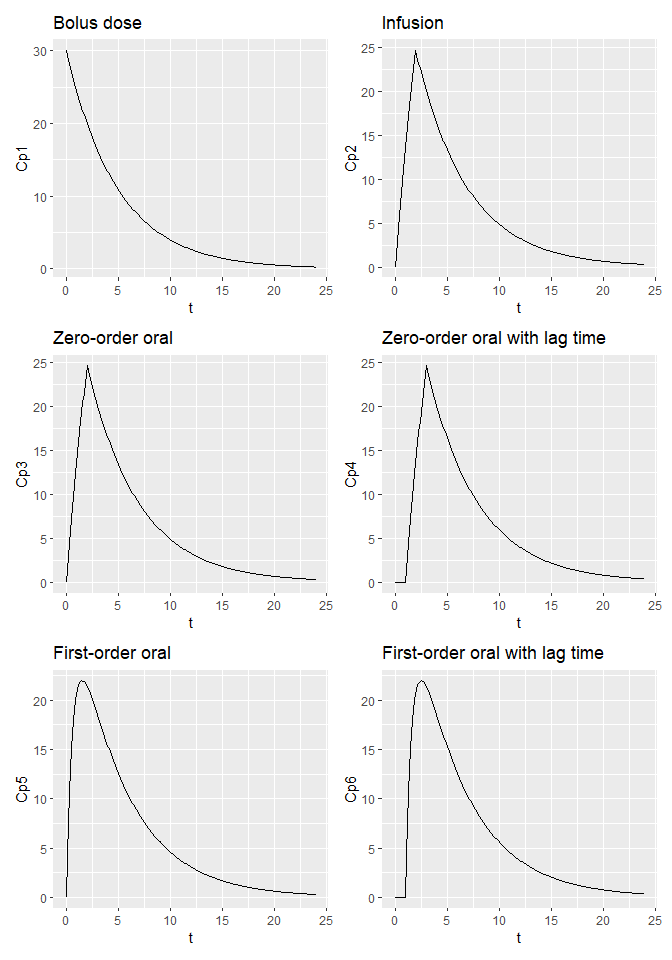Available functions include:

Model Variant Call
3-compartment Single-dose, bolus calc_ss_3cmt_linear_bolus(tad, tau, dose, CL, V1, V2, V3, Q2, Q3)
Single-dose, infusion calc_ss_3cmt_linear_infusion(tad, tau, dose, CL, V1, V2, V3, Q2, Q3, tinf)
Single-dose, zero-order oral absorption calc_ss_3cmt_linear_oral_0(tad, tau, dose, CL, V1, V2, V3, Q2, Q3, dur)
Single-dose, zero-order oral absorption with lag time calc_ss_3cmt_linear_oral_0_lag(tad, tau, dose, CL, V1, V2, V3, Q2, Q3, dur, tlag)
Single-dose, first-order oral absorption calc_ss_3cmt_linear_oral_1(tad, tau, dose, CL, V1, V2, V3, Q2, Q3, ka)
Single-dose, first-order oral absorption with lag time calc_ss_3cmt_linear_oral_1_lag(tad, tau, dose, CL, V1, V2, V3, Q2, Q3, ka, tlag)

For example -


t <- seq(0, 24, by=0.1)

df3ss <- data.frame(t = t,
Cp1 = calc_ss_3cmt_linear_bolus(tad = t, tau = 24, dose = 600, CL = 1, V1 = 20, V2 = 500, V3 = 200, Q2 = 0.5, Q3 = 0.05),
Cp2 = calc_ss_3cmt_linear_infusion(tad = t, tau = 24, dose = 600, CL = 1, V1 = 20, V2 = 500, V3 = 200, Q2 = 0.5, Q3 = 0.05, tinf=2),
Cp3 = calc_ss_3cmt_linear_oral_0(tad = t, tau = 24, dose = 600, CL = 1, V1 = 20, V2 = 500, V3 = 200, Q2 = 0.5, Q3 = 0.05, dur=2),
Cp4 = calc_ss_3cmt_linear_oral_0_lag(tad = t, tau = 24, dose = 600, CL = 1, V1 = 20, V2 = 500, V3 = 200, Q2 = 0.5, Q3 = 0.05, dur=2, tlag=1.5),
Cp5 = calc_ss_3cmt_linear_oral_1(tad = t, tau = 24, dose = 600, CL = 1, V1 = 20, V2 = 500, V3 = 200, Q2 = 0.5, Q3 = 0.05, ka=1.5),
Cp6 = calc_ss_3cmt_linear_oral_1_lag(tad = t, tau = 24, dose = 600, CL = 1, V1 = 20, V2 = 500, V3 = 200, Q2 = 0.5, Q3 = 0.05, ka=1.5, tlag=1.5))

p3.1ss <- ggplot(df3ss, aes(t, Cp1)) +
geom_line() +
labs(title="Bolus dose")

p3.2ss <- ggplot(df3ss, aes(t, Cp2)) +
geom_line() +
labs(title="Infusion")

p3.3ss <- ggplot(df3ss, aes(t, Cp3)) +
geom_line() +
labs(title="Zero-order oral")

p3.4ss <- ggplot(df3ss, aes(t, Cp4)) +
geom_line() +
labs(title="Zero-order oral with lag time")

p3.5ss <- ggplot(df3ss, aes(t, Cp5)) +
geom_line() +
labs(title="First-order oral")

p3.6ss <- ggplot(df3ss, aes(t, Cp6)) +
geom_line() +
labs(title="First-order oral with lag time")

p3.1ss + p3.2ss + p3.3ss + p3.4ss + p3.5ss + p3.6ss  + plot_layout(nrow=3)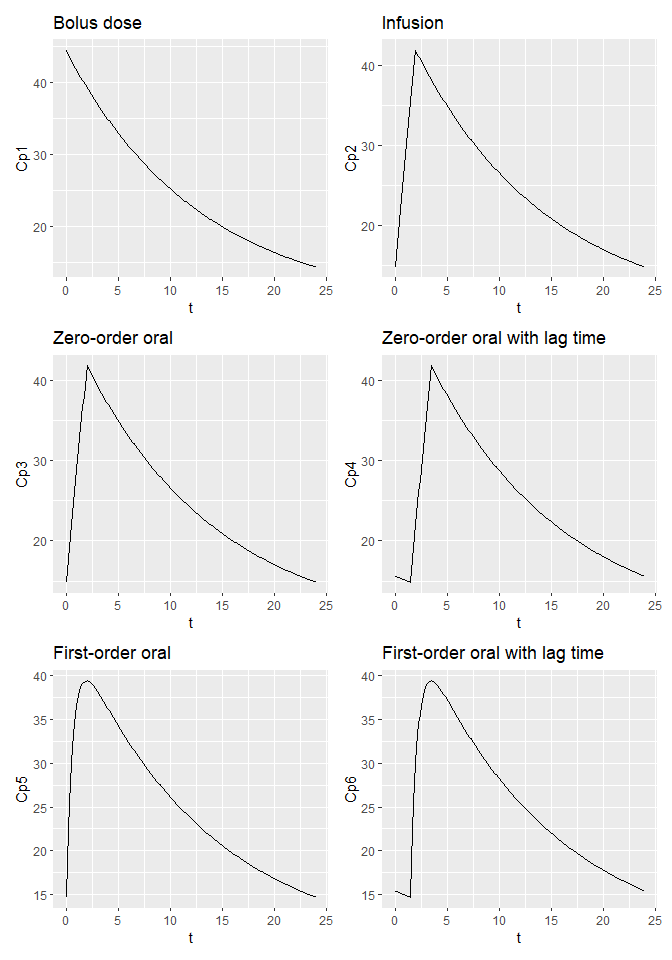## Multiple dosing

Being able to display single-dose and steady state curves for various commonly-seen systems is all very well but it is often more useful to show a concentration-time curve which demonstrates accumulation and time to steady state. The pk_curve() function makes this straightforward by using the principle of superposition.


dfcurve1 <- pk_curve(t=seq(0,168,by=0.1), model="3cmt_oral", ii=24, addl=12,
params=list(CL=1.5, V1=25, V2=2, V3=5, Q2=0.5, Q3=0.25, ka=1))

ggplot(dfcurve1, aes(t, cp)) +
geom_line()